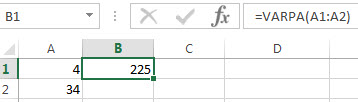# Excel VARPA Function

This post will guide you how to use the VARPA function with syntax and examples in Microsoft excel.

### Description

The Excel VARPA function returns the variance of a population based on an entire population of numbers, text, or logical values. The variance is used to identify the amount that the values vary from the average value across a set of values. And if you want to calculate the variance for a sample of the population, you can use the VARA function.

The VARPA function is a build-in function in Microsoft Excel and it is categorized as a Statistical Function.

The VARPA function is available in Excel 2016, Excel 2013, Excel 2010, Excel 2007, Excel 2003, Excel XP, Excel 2000, Excel 2011 for Mac.

### Syntax

The syntax of the VARPA function is as below:

`= VARPA (value1, [value2], ..)`

Where the VARA function arguments are:

• Value1-This is a required argument.  The first number, text or logical value or cell reference in a sample of a population.
• Value2 -This is an optional argument.  Value arguments 2 to 255 corresponding to a sample of a population. And it can only accept up to 30 number arguments in Excel 2003 or older.

Note:

• Arguments can be the following: numbers; names, arrays, or references that contain numbers; text representations of numbers; or logical values, such as TRUE and FALSE, in a reference.
• Arguments that contain TRUE evaluate as 1; arguments that contain text or FALSE evaluate as 0 (zero).
• The VARPA function is only used for its arguments are an entire population. and if the argument represents a sample of the population, you need to use the VARA function to calculate the variance.
• Arguments that are error values or text that cannot be translated into numbers cause errors.
• If the argument contain the logical values and text representations of numbers and those values will be counted.

### Excel VARPA Function Examples

The below examples will show you how to use Excel VARPA Function to calculate the variance for data that represents the entire population.

1# to get variance of an entire population of data, using the following formula:

=VARPA (A1:A2)### Related Functions

• Excel VARA Function
The Excel VARA function returns the variance of a population based on a sample of numbers, text, or logical values. The syntax of the VARA function is as below:= VARA (value1, [value2], ..)…
• Excel VAR Function
The Excel VAR function returns the variance of a population based on a sample of numbers. This function has been replaced by the VAR.S function in Excel 2010. The syntax of the VAR function is as below:=VAR(number1,[number2],…)…
• Excel VARP Function
The Excel VARP function returns the variance of a population based on an entire population of numbers. The syntax of the VARP function is as below:=VARP(number1,[number2],…)…